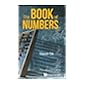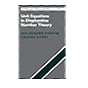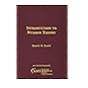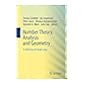Normal view MARC view ISBD view

# Unit equations in Diophantine number theory / Jan-Hendrik Evertse, Universiteit Leiden, Kálmán Györy, Debreceni Egyetem, Hungary.

Material type:TextSeries: Cambridge studies in advanced mathematics: 146.Publisher: Cambridge, United Kingdom : Cambridge University Press, 2015Description: xv, 363 pages ; UKP 49.99 24 cm.Content type: text Media type: unmediated Carrier type: volumeISBN: 9781107097605; 1107097606.Subject(s): Diophantine equations | Diophantine equationsDDC classification: 512.7
Contents:
Part 1. Preliminaries. Basic algebraic number theory -- Algebraic function fields -- Tools from Diophantine approximation and transcendence theory -- Part 2. Unit equations and applications. Effective results for unit equations in two unknowns over number fields -- Algorithmic resolution of unit equations in two unknowns -- Unit equations in several unknowns -- Analogues over function fields -- Effective results for unit equations over finitely generated domains -- Decomposable form equations -- Further applications.
List(s) this item appears in: 2017-10-24
Item type Current location Call number Status Date due Barcode Item holds
Book Chennai Mathematical Institute
General Stacks
512.7 EVE (Browse shelf) Available 10212
Total holds: 0
##### Browsing Chennai Mathematical Institute Shelves , Shelving location: General Stacks Close shelf browserNo cover image available No cover image available512.7 CAI The book of numbers / 512.7 DIE Arithmetic and geometry / 512.7 EDW Higher arithmetic : an algorithmic introduction to number theory / 512.7 EVE Unit equations in Diophantine number theory / 512.7 FLA Introduction to number theory / 512.7 GOL Automorphic representations and L-functions for the general linear group /Vol II 512.7 GOL Number theory, analysis and geometry : in memory of Serge Lang /

Includes bibliographical references (pages 337-357) and index.

Part 1. Preliminaries. Basic algebraic number theory -- Algebraic function fields -- Tools from Diophantine approximation and transcendence theory -- Part 2. Unit equations and applications. Effective results for unit equations in two unknowns over number fields -- Algorithmic resolution of unit equations in two unknowns -- Unit equations in several unknowns -- Analogues over function fields -- Effective results for unit equations over finitely generated domains -- Decomposable form equations -- Further applications.